## The Spatial Matrix Process Effect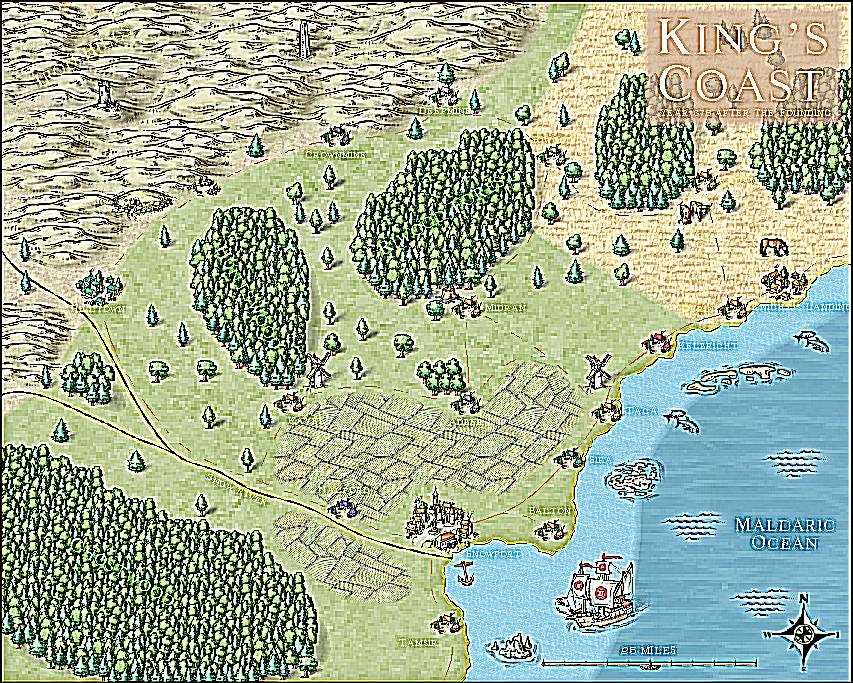One of the most powerful effects in CC3+ is also the least used one. And that is kind of understandable, because it is also one of the more complicated ones. So in this article, I’m going to give you a bit of an introduction to the Spatial Matrix Process (SMP) effect (Not to be confused with the RGB Matrix Effect).

SMP is a custom filter where you have a lot of control over what the effect will do, it doesn’t have a pre-defined purpose like the other effects, but can be used to create a variety of different effects. Note that some of the results you can accomplish using this effect is already implemented as separate effects, such as blur.

All of the examples in this article uses the effect as a Whole Map effect, and I use the standard tutorial map from the user manual as an example, which you find in the @Tutorials\UserManual folder.

## Presets

The configuration of this effect is rather complex, so I recommend you start out by looking at the various presets. You can access these by clicking the Load button in the dialog, and browse to @Filters\Settings. Using these, you can see how entering numbers in all those boxes can affect your map.## Explaining the Effect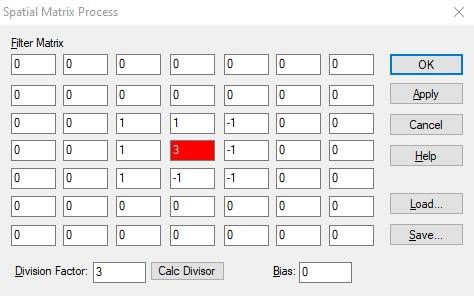So, how does this effect actually work? All those boxes may look confusing, but what you are seeing here is simply a method of calculating the color value of the current pixel depending on the color values of the surrounding pixels. The center box, colored red here, represents the current pixel, while all the other boxes represents the neighboring pixels around it. This effect process every single pixel in the image, one by one, so every pixel gets their turn being the “red one” in the middle. And for each pixel processed in this way, it is affected by the pixels around it. The values you put in the boxes are relative values, telling how much they should be counted. Positive values brightens the result, negative values darkens it.And any pixels with a 0 don’t contribute to the outcome. This allows you to not care about every pixel in that 7×7 grid. For example, the Emboss preset in my image only base the result on the immediately surrounding pixels, the 3×3 box instead of the full 7×7.

### Division Factor

At the bottom of the dialog, you’ll see a division factor input. As I mentioned above, positive values for the pixels brightens the result, while negative values darkens it. But for most images, you actually want the relative brightness to stay the same. However, the only way this will happen is if the final modifications adds up to 1. For some settings they do that naturally, but if not, you can use the division factor to even this out. And this is quite simple. If you wish to keep the current brightness, the division factor has to be equal to the sum of the modifications in the matrix above. So in my emboss example here, you can see that the values sum up to 3 (1 + 1 + -1 +1 +3 + -1 + 1 + -1 + -1 = 3). So by setting the division factor to 3, brightness is preserved. If it is set to a smaller value, the image will brighten, and a higher value will cause the image to darken. Clicking the Calc Divisor button juts makes CC3+ calculate the appropriate value for keeping the brightness for you. Basically, what happens is the sum of the modifications in the matrix are divided by this value. Even if you wish to darken or lighten the image, to note that the end result of this calculation should still be low number.

### Bias

The bias is added as a final value after the calculation and division. It basically also allow you to brighten/darken the image, but the scale it work on is different since it applies after the division and it provides a fixed modification not dependent on the pixel values. This can be used to compensate for a calculation that doesn’t give 1 as a result.

## A Few Examples

So, let us just do a few examples and explain the numbers

### Lighten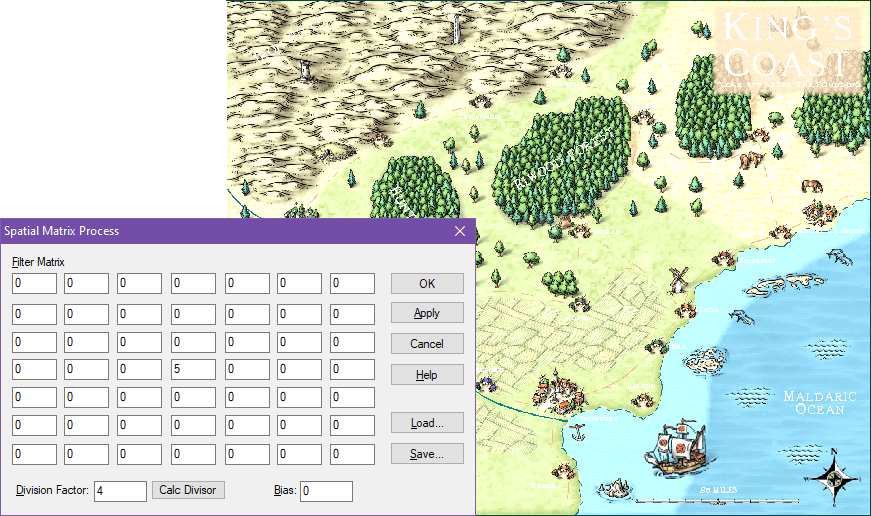So, let us start by a very simple example that lightens the image without changing it. I’ve set the current pixel to 5 and the division factor to 4. Since none of the other pixels have a value, they won’t have any effect on the final calculation, but since the total sum of the matrix (5) is higher than the division factor(4), it results in each pixel of the image being brightened just a bit. Remember, if the final value is larger than 1, we end up with a brighter image than we started with.

### Darken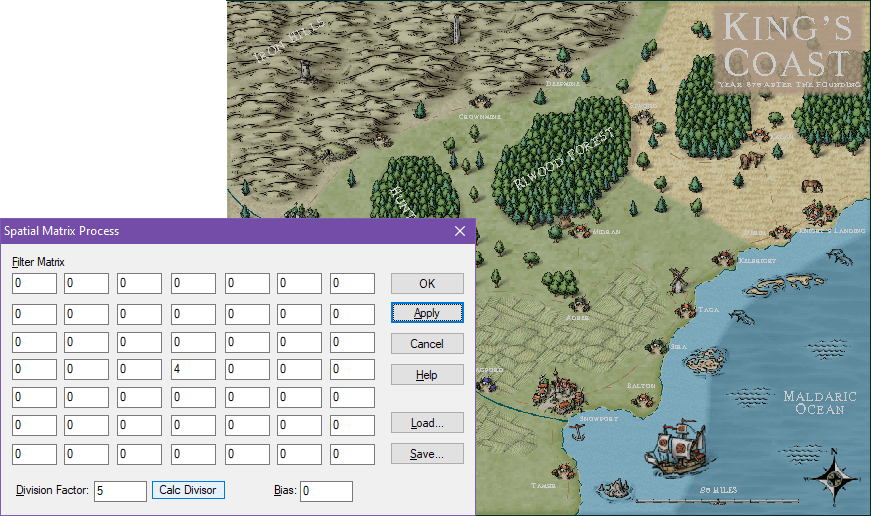Similarly, darkening the image is equally simple. Here, I’ve done the exact same thing as above, just swapped the values. Since the sum of the matrix is now 4, divided by the factor of 5, we end up with a result lower than 1, which will serve to darken the image. Remember that these values are relative values, so using 8 and 10 or 400 and 500 for the values will end up with the same result.

### SharpenA positive value for the active pixel surrounded by negative values will result in a sharpening effect. For my example image here, look at the forests. The left one is sharpened, while the right one is using the default symbol appearance. I pushed the effect a bit hard here to make it easier to see in my images (you’ll probably still want to click the image to see it in full size), but these effects can often work better when used to make a more subtle result.

But, if you really want, you can take it to the next level by increasing the values a bit, like in my example here.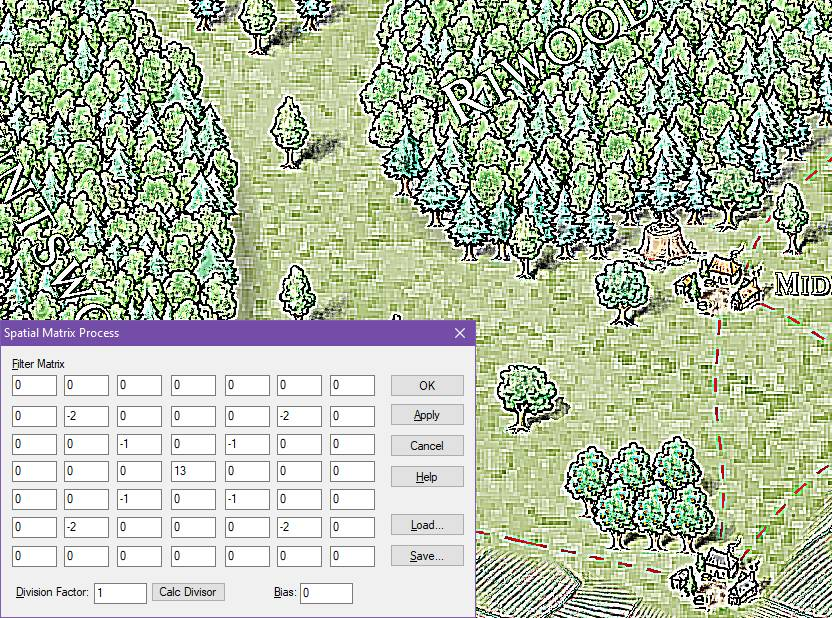With this much sharpening applied, it can be difficult to even identify Mike Schley’s original style in the artwork.

Probably not a setting you will use for your daily mapping, but it can create an interesting variation for special needs.  And you can of course make the effect even stronger. Combining it with a Division factor that is slightly off the 1-mark can also give interesting results here.

### BlurBlur is one of the basic features filters like these are used for. Blurring is easily accomplished by using  a value of 1 for the central pixel, and bringing in values from the surrounding pixels. A subtle blur can be accomplished by just adding 1 for the four bordering pixels, but I’ve taken it a bit further for my example here to more clearly show the result. Again, click the image to see the larger version to appreciate the effect properly.

### Edge Detect

How about a strong edge detect?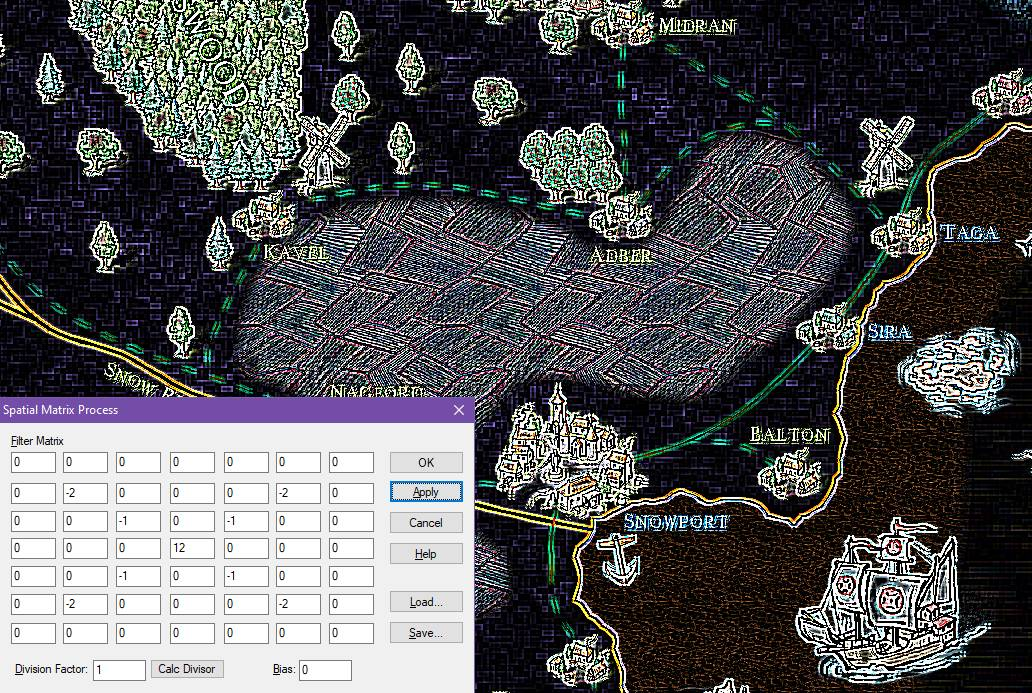Edge detect settings are used to find edges in the image, and make them visible. In this example, this is done by using a positive value for the center pixel, and negative values for surrounding pixels, which sums to 0. The division factor was left at 1. This results in generally dark image where the edges are highly visible.